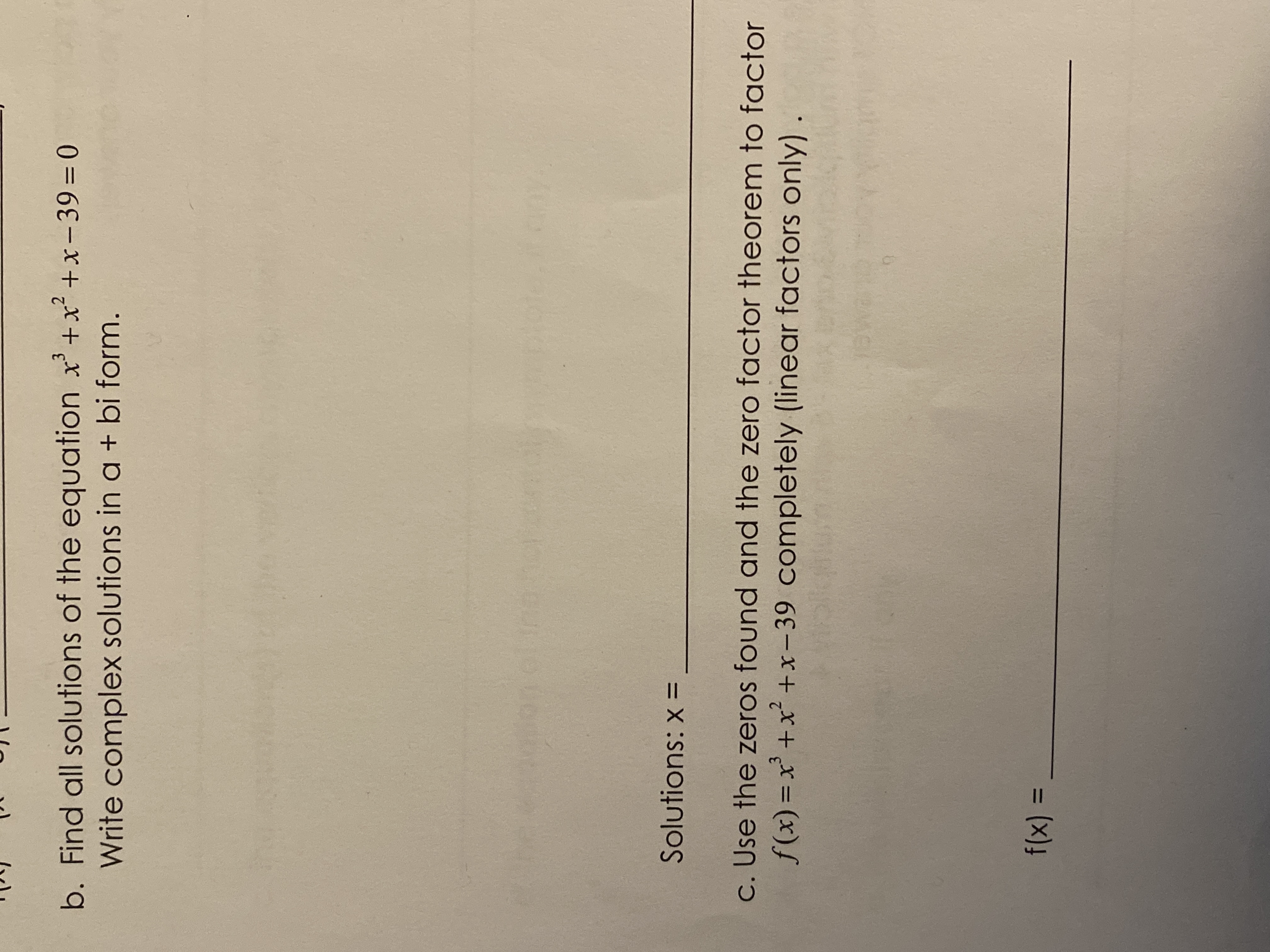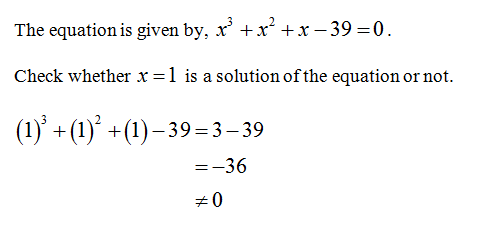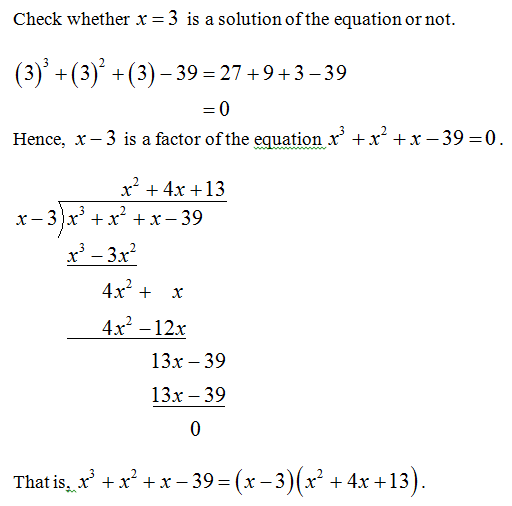# b. Find all solutions of the equation x' +x² +x-39 = 0Write complex solutions in a + bi form.Solutions: x =c. Use the zeros found and the zero factor theorem to factorf(x) =x' +x² +x-39 completely (linear factors only) .f(x) =%3D

Question
1 views

B.help_outlineImage Transcriptioncloseb. Find all solutions of the equation x' +x² +x-39 = 0 Write complex solutions in a + bi form. Solutions: x = c. Use the zeros found and the zero factor theorem to factor f(x) =x' +x² +x-39 completely (linear factors only) . f(x) = %3D fullscreen
check_circle

Step 1Step 2...

### Want to see the full answer?

See Solution

#### Want to see this answer and more?

Solutions are written by subject experts who are available 24/7. Questions are typically answered within 1 hour.*

See Solution
*Response times may vary by subject and question.
Tagged in